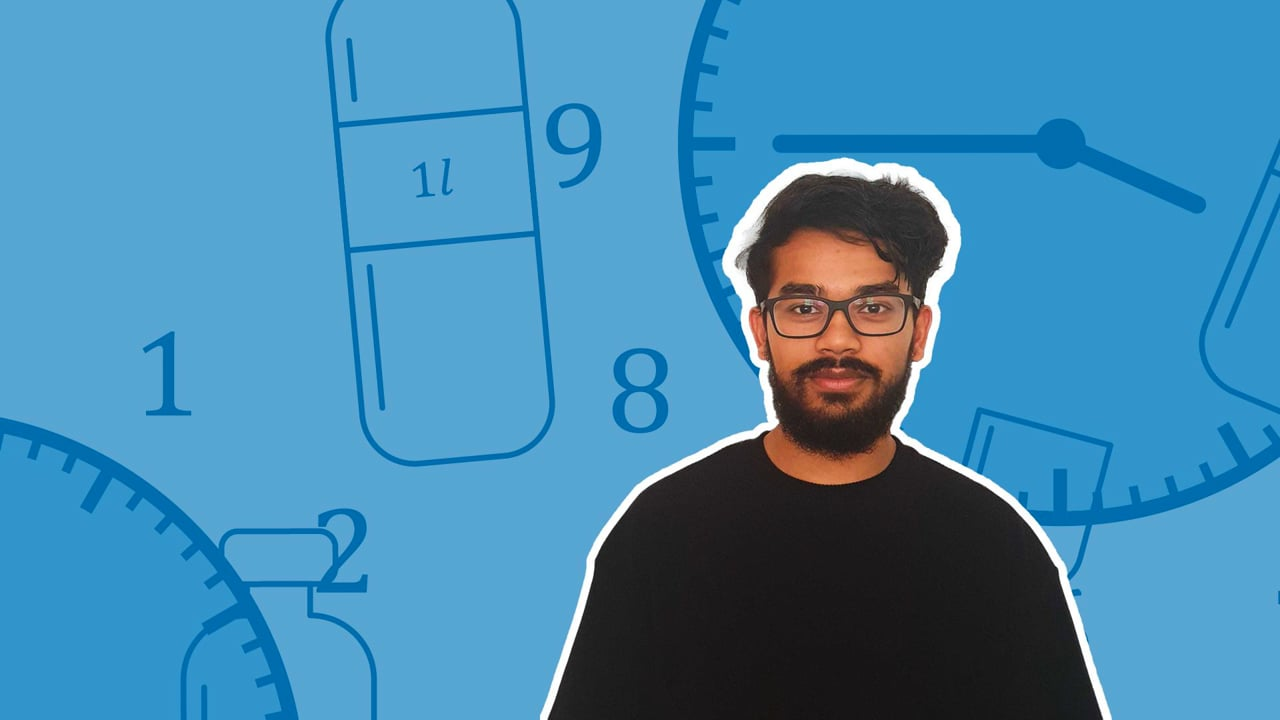Chapter overviewMaths

Number and place value

Multiplication and division

Fractions

Measurement

Geometry - properties of shapes

Geometry - position and direction

Statistics

Maths

# Converting time between analogue and digital clocks0%

Summary

# Converting time between analogue and digital clocks

## ​​In a nutshell

What time it is can be described in $3$ ways. Analogue clocks use $3$ different hands around a dial to show how far into the day it is, while digital clocks use separated pairs of numbers to describe the hour, minute and second. The final way the time is described is using English, like how you would tell someone the time in conversation.

## A.M. and P.M.

When talking about time, there are two periods: A.M. and P.M. These are used in $12$-hour time to distinguish between the morning and the afternoon/evening.​

### Definitions

#### hOURS

​​A.M.

Before midday

Ante Meridiem

$00:00-11:59$​​

P.M.

After midday

Post Meridiem

$12:00-23:59$​​

### Convert between 24-hour and 12-hour time

• A.M. - The hours remain the same.
• P.M. - Minus $12$.

### Convert between 12-hour and 24-hour time

• ​A.M. - The hours remain the same.
• P.M. - Add on $12$.
##### Example 1

What is $2:15$ P.M. in $24$-hour time?

$2+12=14$

Rewrite in $24$-hour time.

The time is $\underline{14:15}$.

​​

## Analogue to digital conversion

Analogue clocks use $12$-hour time, which means you may need to convert to $24$-hour time for its digital equivalent.

#### Procedure

 1 Convert the analogue time into the English phrasing. 2 Split the English phrasing into the hour, minute and seconds. 3 Write each in digital format, converting to $24$-hour time if required.

##### Example 2

Write the time of the following analogue clock in $24$-hour digital form, given that the time was taken in the evening:

Convert to English phrasing.

It is thirty-eight minutes and fifteen seconds past eleven in the evening.

Split into hours, minutes and seconds.

Current hour: $11$​​

Current minute: $38$​​

Current seconds: $15$​​

Convert from $12$-hour time to $24$​-hour time.

$11+12=23$​​

Rewrite in digital terms.

The time is $\underline{23:38:15}$.

Note: If you're not asked to use $12$-hour time, make sure to include A.M. or P.M. in your answer!​

## Digital to analogue conversion

#### ​​procedure

 1 Draw the second hand on the clock face (if required). 2 Draw the minute hand on the clock face pointing towards the current minute. 3 Locate the current hour on the clock face. Draw the hour hand so that it lies $\dfrac{\text{current minute}}{60}$  of the way between the current hour and next.

Tip: Remember, the hour hand should be short, the minute hand long and the seconds hand should be long and thinner than the minute hand.

##### Example 3

Draw the minute and hour hand of an analogue clock when the time is $20:30$​.

The minute hand points towards $6$ as this shows it is $30$ minutes past the hour.

Work out the current hour and convert to $12$-hour time.

$20 - 12 = 8$.

Decide where the hour hand should point.

$\dfrac{30}{60}=\dfrac{1}{2}$

The hour hand points half way between $8$​ and $9$.

Hence the analogue clock has hands as follows:

FAQs

• Question: What do A.M. and P.M. mean?

Answer: A.M. stands for Ante Meridiem, which means before midday. P.M. stands for Post Meridiem, which means after midday.

• Question: How do I convert from analogue to digital time?

Answer: To convert from analogue to digital time: 1. Convert the analogue time into the English phrasing. 2. Split the English phrasing into the hour, minute and seconds. 3. Write each in digital format, converting to 2424-hour time if required.

• Question: How do I convert from digital to analogue time?

Answer: To convert from digital to analogue time: 1. Draw the second hand on the clock face (if required). 2. Draw the minute hand on the clock face pointing towards the current minute. 3. Locate the current hour on the clock face. Draw the hour hand so that it lies current minute/60 of the way between the current hour and next.

Theory

Exercises